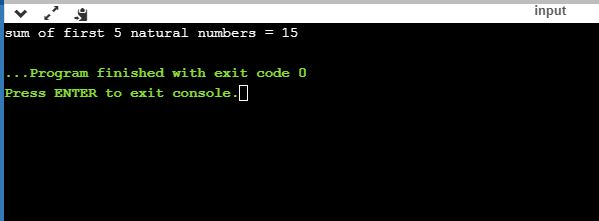UncleCoder.com

UncleCoder.com

Free programming examples and instructions

# C program to study do-while loop

## C program to study do-while loop

by Krishna

Posted on 27 Jul 2018 Category: C Views: 1334

C program to study do-while loop

The 'do-while' loop is similar to the while loop with one important difference. The body of 'do-while' loop is executed at least once.  Only then, the test expression is evaluated.

The syntax of the 'do-while' loop is:

``````do
{
// statements inside the body of the loop
}
while (testExpression);``````

The body of do...while loop is executed once. Only then, the test expression is evaluated. If the test expression is true, the body of the loop is executed again and the test expression is evaluated. This process goes on until the test expression becomes false. If the test expression is false, the loop ends.

``````#include <stdio.h>
int main ()
{
int sum = 0, i = 1;  //initialization of counter variable i
do
{
sum = sum+i;
i++;  //increment of counter variable
}while(i <= 5);  //coondition of do while
printf("sum of first 5 natural numbers = %d",sum);
return 0;
} ``````

OUTPUT